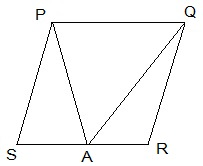Guru

# A farmer was having a field in the form of a parallelogram PQRS. She took any point A on RS and joined it to points P and Q. In how many parts the fields is divided? What are the shapes of these parts? The farmer wants to sow wheat and pulses in equal portions of the field separately. How should she do it? Q.6

• -1

Please give me the best way for solving the problem of class 9th ncert math of Areas of Parallelograms and Triangles chapter of math of class 9th of exercise 9.2of question no 6  what is the tricky way for solving this question A farmer was having a field in the form of a parallelogram PQRS. She took any point A on RS and joined it to points P and Q. In how many parts the fields is divided? What are the shapes of these parts? The farmer wants to sow wheat and pulses in equal portions of the field separately. How should she do it?

Share

1.The field is divided into three parts each in triangular shape.

Let, ΔPSA, ΔPAQ and ΔQAR be the triangles.

Area of (ΔPSA + ΔPAQ + ΔQAR) = Area of PQRS — (i)

Area of ΔPAQ = ½ area of PQRS — (ii)

Here, the triangle and parallelogram are on the same base and in-between the same parallel lines.

From (i) and (ii),

Area of ΔPSA +Area of ΔQAR = ½ area of PQRS — (iii)

From (ii) and (iii), we can conclude that,

The farmer must sow wheat or pulses in ΔPAQ or either in both ΔPSA and ΔQAR.

• 0